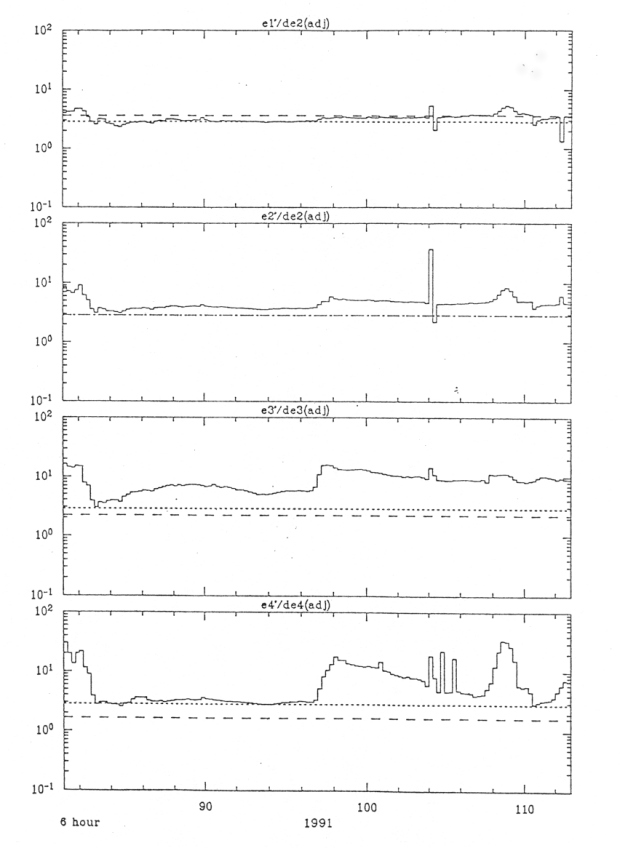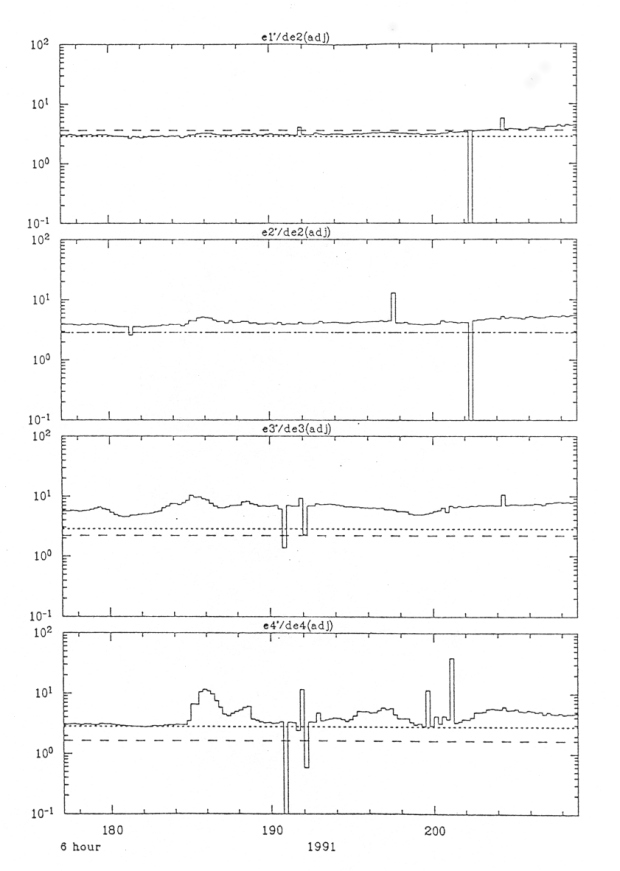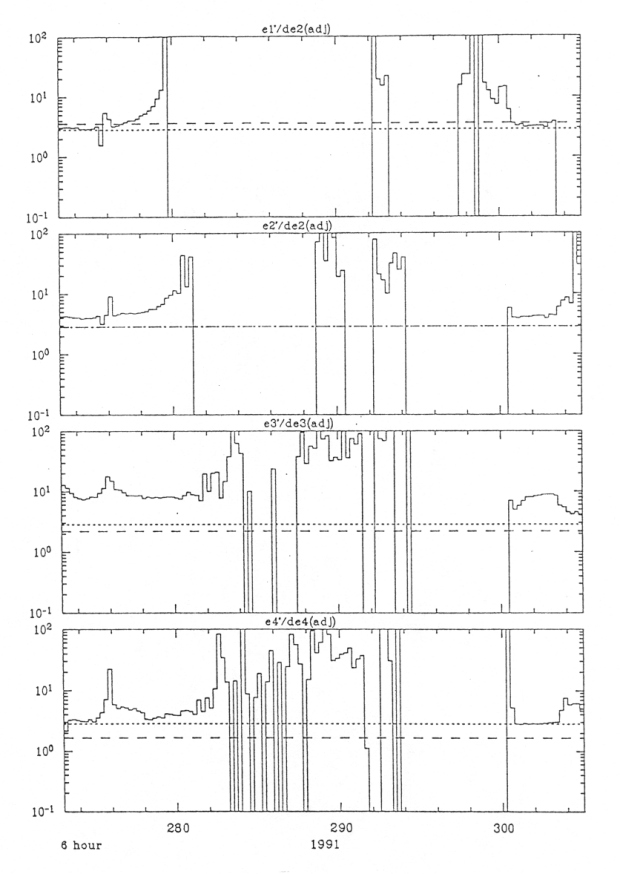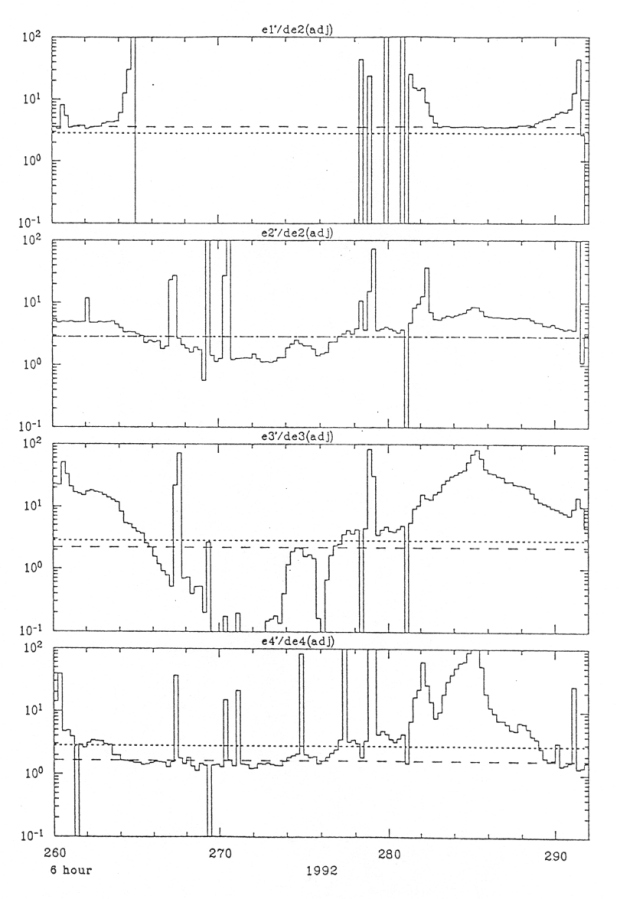ULYSSES

Ulysses HISCALE Data Analysis Handbook

4.9 Modeling of the Magnetic Electron Deflection System

4.9.1 Without Scattering

Figure 4.77a Ratio plots for all four energy channels for a 32-day period. The dotted line represents the predicted ratio for IDF.DAT values of G, whereas the dashed line represents the predicted ratio for Table 4.18 values of G.Figure 4.77b Ratio plots for a later time period in 1991. Note how the ion event around day 186 affects the ratio.Figure 4.77c Ratio plots including the electron event around day 301.Figure 4.77d Ratio plots for a 32-day period in 1992. Note that now it appears the Table 4.19 values give better results.